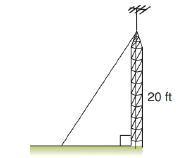Chapter 5.4, Problem 15EElementary Geometry For College St...

7th Edition
Alexander + 2 others
ISBN: 9781337614085

Solutions

Chapter
SectionElementary Geometry For College St...

7th Edition
Alexander + 2 others
ISBN: 9781337614085
Textbook Problem

A guy wire 25 ft long supports an antenna at a point that is 20 ft above the base of the antenna. How far from the base of the antenna is the guy wire secured?To determine

To Find:

Length from the base of the antenna to the guy wired secured.

Explanation

Given A guy wire 25 ft. long supports an antenna at a point that is 20 ft above the base of the antenna.

Let x be the length from the base of the antenna to the guy wired secured.

From the figure it is in form of right triangle in which hypotenuse = 25 ft., and one of its side is 20 ft.

From the given data,

252=202+x2625

Still sussing out bartleby?

Check out a sample textbook solution.

See a sample solution

The Solution to Your Study Problems

Bartleby provides explanations to thousands of textbook problems written by our experts, many with advanced degrees!

Get Started

Sketch the graph of the function defined by f(x)={x+1ifx1x2+4x1ifx1

Applied Calculus for the Managerial, Life, and Social Sciences: A Brief Approach

Find all possible real solutions of each equation in Exercises 3144. x327=0

Finite Mathematics and Applied Calculus (MindTap Course List)

Differentiate the function. R(a) = (3a + 1)2

Single Variable Calculus: Early Transcendentals, Volume I

WRITING ABOUT CONCEPTS Using Substitution Describe why x(5x2)3dxu3du Where u=5x2.

Calculus: Early Transcendental Functions (MindTap Course List)

True or False: x2x24dx should be evaluated using integration by parts.

Study Guide for Stewart's Single Variable Calculus: Early Transcendentals, 8th